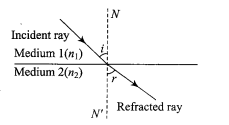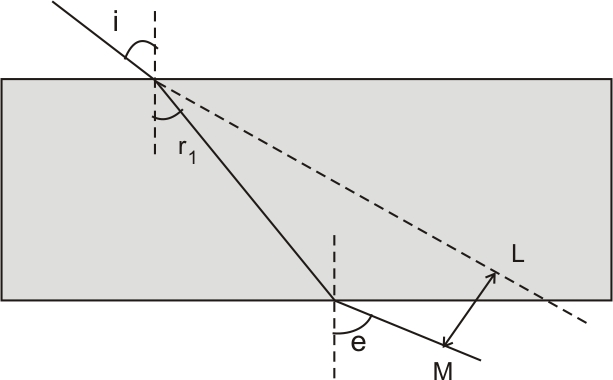#### Write laws of refraction. Explain the same with the help of ray diagram, when a ray of light passes through a rectangular glass slab.Laws of refraction:

1. The incident ray, the normal to any refracting surface at the point of incidence, and the refracted ray all lie in the same plane called the plane of incidence or plane of refraction.
2. The ratio of the sine of the angle of incidence to the angle of refraction is always constant.or= refractive index of the second medium with respect to the first medium.

For a glass slab as shown in figurei = angle of incidence

r1 = angle of reflection